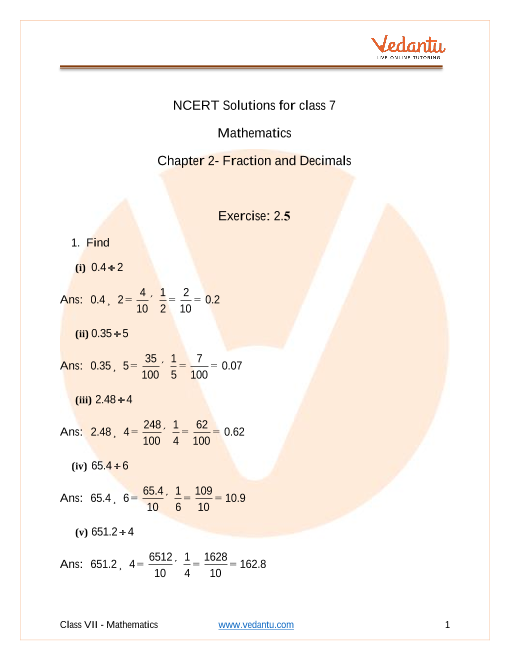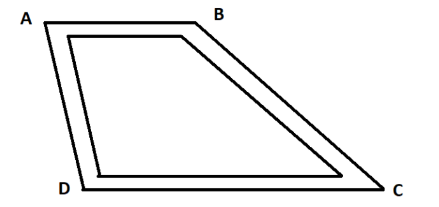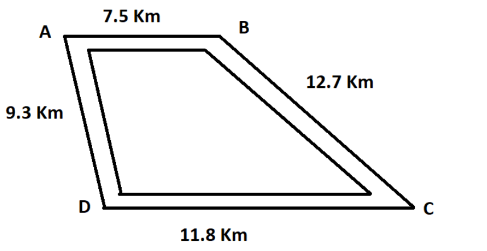# NCERT Solutions for Class 7 Maths Chapter 2 Fractions and Decimals (EX 2.5) Exercise 2.5

## NCERT Solutions for Class 7 Maths Chapter 2 Fractions and Decimals (EX 2.5) Exercise 2.5

Free PDF download of NCERT Solutions for Class 7 Maths Chapter 2 Exercise 2.5 (EX 2.5) and all chapter exercises at one place prepared by expert teacher as per NCERT (CBSE) books guidelines. Class 7 Maths Chapter 2 Fractions and Decimals Exercise 2.5 Questions with Solutions to help you to revise complete Syllabus and Score More marks. Register and get all exercise solutions in your emails. Every NCERT Solution is provided to make the study simple and interesting on Vedantu. Subjects like Science, Maths, English will become easy to study if you have access to NCERT Solution for Class 7 Science , Maths solutions and solutions of other subjects.

Do you need help with your Homework? Are you preparing for Exams?
Study without Internet (Offline)Book your Free Demo session
Get a flavour of LIVE classes here at VedantuVedantu Improvement Promise
We promise improvement in marks or get your fees back. T&C Apply*

## Access NCERT Solutions for Class 7 Maths Chapter 2 - Fractions and Decimals

Exercise - 2.5

1. Which is greater:

(i) $\text{0}\text{.5}\,\text{or}\,\text{0}\text{.05}$

Ans: Finding the greater term, $0.5>0.05$ .

(ii) $\text{0}\text{.7}\,\text{or}\,\text{0}\text{.5}$

Ans: Finding the greater term, $0.7>0.5$ .

(iii) $\text{7 or 0}\text{.7}$

Ans: Finding the greater term, $7>0.7$ .

(iv) $\text{1}\text{.37 or 1}\text{.49}$

Ans: Finding the greater term, $1.37<1.49$ .

(v) $\text{2}\text{.03 or 2}\text{.30}$

Ans: Finding the greater term, $2.03<2.30$ .

(vi) $\text{0}\text{.8 or 0}\text{.88}$

Ans: Finding the greater term, $0.8<0.88$ .

2. Express as rupees using decimals:

(i) $\text{7}\,\text{paise}$

Ans: Expressing the term as rupees,

$\text{7}\,\text{paise=Re}\text{.}\dfrac{\text{7}}{\text{100}}\text{=Re}\text{.0}\text{.07}$

(ii) $\text{7 rupees 7 paise}$

Ans: Expressing the term as rupees,$\text{7}\,\text{rupees}\,\,\text{7}\,\,\text{paise=Rs}\text{.7+Re}\text{.}\dfrac{\text{7}}{\text{100}}\text{=Rs}\text{.7+Rs}\text{.0}\text{.07=Rs}\text{.7}\text{.07}$

(iii) $\text{77 rupees 77 paise}$

Ans: Expressing the term as rupees,$\text{77}\,\text{rupees}\,\,\text{77}\,\,\text{paise=Rs}\text{.77+Re}\text{.}\dfrac{\text{77}}{\text{100}}\text{=Rs}\text{.77+Rs}\text{.0}\text{.77=Rs}\text{.77}\text{.77}$

(iv) $\text{50 paise}$

Ans: Expressing the term as rupees,

$\text{50}\,\text{paise=Re}\text{.}\dfrac{\text{50}}{\text{100}}\text{=Re}\text{.0}\text{.50}$

(v) $\text{235 paise}$

Ans: Expressing the term as rupees,

$\text{235}\,\text{paise=Re}\text{.}\dfrac{\text{235}}{\text{100}}\text{=Rs}\text{.2}\text{.35}$

3. Find

(i) Express $\text{5}$ cm in metre and kilometer.

Ans: Expressing $5$ cm in meter and kilometer,

$\because \,\,\text{100}\,\text{cm=1}\,\text{meter}$

$\therefore \,\,\text{1}\,\text{cm=}\dfrac{\text{1}}{\text{100}}\,\text{meter}\Rightarrow \text{5}\,\text{cm=}\dfrac{\text{5}}{\text{100}}\text{=0}\text{.05}\,\text{meter}$

And, $\because \,\,\text{1000}\,\text{meters=1}\,\text{kilometers}$

$\therefore \,\,\text{1}\,\text{meter=}\dfrac{\text{1}}{\text{1000}}\,\text{kilometers}$ $\Rightarrow \text{0}\text{.05}\,\text{meter=}\dfrac{\text{0}\text{.05}}{\text{1000}}\text{=0}\text{.00005}\,\,\text{kilometer}$

(ii) Express $\text{35}$ mm in cm, m and km.

Ans: Expressing $35$ mm in cm, m and km.

$\because \,\,\text{10}\,\text{mm=1}\,\text{cm}$

$\therefore \,\,\text{1}\,\text{mm=}\dfrac{\text{1}}{\text{10}}\,\text{cm}\Rightarrow \text{35}\,\text{mm=}\dfrac{\text{35}}{\text{10}}\text{=3}\text{.5}\,\text{cm}$

And, $\because \,\,\text{100}\,\text{cm=1}\,\text{meter}$

$\therefore \,\,\,\text{1}\,\text{cm=}\dfrac{\text{1}}{\text{100}}\,\text{meter}\Rightarrow \text{3}\text{.5}\,\text{cm=}\dfrac{\text{3}\text{.5}}{\text{100}}\text{=0}\text{.035}\,\text{meter}$

Also, $\because \,\,\text{1000}\,\text{meters=1}\,\text{kilometers}$

$\therefore \,\,\text{1}\,\text{meter=}\dfrac{\text{1}}{\text{1000}}\,\text{kilometer}$ $\Rightarrow \,\,\text{0}\text{.035}\,\text{meter=}\dfrac{\text{0}\text{.035}}{\text{1000}}\text{=0}\text{.000035}\,\text{kilometer}$

4. Express in kg:

(i) $\text{200}\,\text{g}$

Ans: Converting from grams to kilograms,

$\text{200}\,\text{g=}\left( \text{200 }\!\!\times\!\!\text{ }\dfrac{\text{1}}{\text{1000}} \right)\,\text{kg=0}\text{.2}\,\text{kg}$

(ii) $\text{3470}\,\text{g}$

Ans: Converting from grams to kilograms,

$3470\,\text{g=}\left( \text{3470 }\!\!\times\!\!\text{ }\dfrac{\text{1}}{\text{1000}} \right)\,\text{kg=3}\text{.470}\,\text{kg}$

(iii) $\text{4}\,\text{kg}\,\text{8}\,\text{g}$

Ans: Converting from grams to kilograms,$\text{4}\,\text{kg}\,\text{8}\,\text{g=4}\,\text{kg}\,\text{+}\left( \text{8 }\!\!\times\!\!\text{ }\dfrac{\text{1}}{\text{1000}} \right)\,\text{kg=4}\,\text{kg+0}\text{.008}\,\text{kg=4}\text{.008}\,\text{kg}$

5. Write the following decimal numbers in the expanded form:

(i) $\text{20}\text{.03}$

Ans: Converting the decimal number in expanded form,

$20.03=2\times 10+0\times 1+0\times \dfrac{1}{10}+3\times \dfrac{1}{100}$

(ii) $\text{2}\text{.03}$

Ans: Converting the decimal number in expanded form, $2.03=2\times 1+0\times \dfrac{1}{10}+3\times \dfrac{1}{100}$

(iii) $\text{200}\text{.03}$

Ans: Converting the decimal number in expanded form, $200.03=2\times 100+0\times 10+0\times 1+0\times \dfrac{1}{10}+3\times \dfrac{1}{100}$

(iv) $\text{2}\text{.034}$

Ans: Converting the decimal number in expanded form, $2.034=2\times 1+0\times \dfrac{1}{10}+3\times \dfrac{1}{100}+4\times \dfrac{1}{1000}$

6. Write the place value of $\text{2}$ in the following decimal numbers:

(i) $\text{2}\text{.56}$

Ans: The place value of $2$ in $2.56$ $=2\times 1=2\,$ ones

(ii) $\text{21}\text{.37}$

Ans: The place value of $2$ in $21.37=2\times 10=2$ tens

(iii) $\text{10}\text{.25}$

Ans: The place value of $2$ in $10.25=2\times \dfrac{1}{10}=2$ tenths

(iv) $\text{9}\text{.42}$

Ans: The place value of $2$ in $9.42=2\times \dfrac{1}{100}=2$ hundredth

(v) $\text{63}\text{.352}$

Ans: The place value of $2$ in $63.352=2\times \dfrac{1}{1000}=2$ thousandth.

7. Dinesh went from place$\text{ }\!\!~\!\!\text{ A}$ to place $\text{B}$ and from there to place$\text{C}$ .

$\text{A}$ is $\text{7}\text{.5}$ km from $\text{B}$ and $\text{B}$ is $\text{12}\text{.7}$ km from$\text{C}$ . Ayub went from place $\text{A}$ to place $\text{D}$ and from there to place$\text{C}$ . $\text{D}$ is $\text{9}\text{.3}$ km from $\text{A}$ and $\text{C}$ is $\text{11}\text{.8}$ km from$\text{D}$ . Who travelled more and by how much?Ans: Given: The distance travelled by Dinesh when he went from place $\text{A}$ to place $\text{B = 7}\text{.5 km}$ and from place$\text{B to C = 12}\text{.7 km}$According to the figure,

The total distance covered by Dinesh $\text{= AB + BC }$

Substituting the values,

$\text{=7}\text{.5 + 12}\text{.7 = 20}\text{.2 km}$

The total distance covered by Ayub $\text{= AD + DC }$

Substituting the values,

$\text{=9}\text{.3 + 11}\text{.8 = 21}\text{.1 km}$

Comparing the total distance covered by Ayub and Dinesh,

$\text{21}\text{.1 km 20}\text{.2 km}$

Hence, Ayub covered $\text{21}\text{.1 -- 20}\text{.2 = 0}\text{.9 km = 900m}$ more distance as compared to Dinesh.

8. Shyam bought $\text{5 kg 300 g}$ apples and $\text{3 kg 250 g}$ mangoes. Sarala bought $\text{4 kg 800 g}$ oranges and $\text{4 kg 150 g}$ bananas. Who bought more fruits?

Ans: Given:

The total weight of fruits bought by Shyam$\text{ = 5 kg 300 g + 3 kg 250 g = 8 kg 550 g}$

And the total weight of fruits bought by Sarala$\text{= 4 kg 800 g + 4 kg 150 g = 8 kg 950 g}$

Comparing the quantity of fruits bought by Shyam and Sarala, $\text{8}\,\text{kg}\,\text{550}\,\text{g8}\,\text{kg}\,\text{950}\,\text{g}$

Observe that quantity of fruits bought by Sarala is greater.

Hence, Sarala bought more fruits than Shyam.

9. How much less is $\text{28 km}$ then $\text{42}\text{.6 km}$ ?

Ans: Given: The two distances are $\text{42}\text{.6}\,\text{km}\,\text{and}\,\text{28}\,\text{km}$

Finding the difference of $\text{42}\text{.6}\,\text{km}\,\text{and}\,\text{28}\,\text{km}$ ,

$\text{42}\text{.6-28}\text{.0=14}\text{.6}\,\text{km}$

Hence, $\text{14}\text{.6}\,\text{km}$ less is $\text{28}\,\text{km}$ then $\text{42}\text{.6}\,\text{km}$ .

## NCERT Solutions for Class 7 Maths Chapter 2 Fractions and Decimals Exercise 2.5

Opting for the NCERT solutions for Ex 2.5 Class 7 Maths is considered as the best option for the CBSE students when it comes to exam preparation. This chapter consists of many exercises. Out of which we have provided the Exercise 2.5 Class 7 Maths NCERT solutions on this page in PDF format. You can download this solution as per your convenience or you can study it directly from our website/ app online.

Vedantu in-house subject matter experts have solved the problems/ questions from the exercise with the utmost care and by following all the guidelines by CBSE. Class 7 students who are thorough with all the concepts from the Maths textbook and quite well-versed with all the problems from the exercises given in it, then any student can easily score the highest possible marks in the final exam. With the help of this Class 7 Maths Chapter 2 Exercise 2.5 solutions, students can easily understand the pattern of questions that can be asked in the exam from this chapter and also learn the marks weightage of the chapter. So that they can prepare themselves accordingly for the final exam.

Besides these NCERT solutions for Class 7 Maths Chapter 2 Exercise 2.5, there are plenty of exercises in this chapter which contain innumerable questions as well. All these questions are solved/answered by our in-house subject experts as mentioned earlier. Hence all of these are bound to be of superior quality and anyone can refer to these during the time of exam preparation. In order to score the best possible marks in the class, it is really important to understand all the concepts of the textbooks and solve the problems from the exercises given next to it.

Do not delay any more. Download the NCERT solutions for Class 7 Maths Chapter 2 Exercise 2.5 from Vedantu website now for better exam preparation. If you have the Vedantu app in your phone, you can download the same through the app as well. The best part of these solutions is these can be accessed both online and offline as well.SHARETWEETSHARESUBSCRIBE